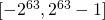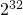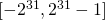# Numeric types in Perl

Dealing with numeric types in Perl is not as strait-forward as in other programming languages. We can use „scalars“ out of the box, but then we get floating point numbers, more precisely what is called „double“ in most programming languages. This is kind of ok for trivial programs, but we should make a deliberate choice on what to use.

Actually the Perl programming language gives us (at least) two more choices. We can use 64-bit integers (or 32-bit on some platforms) by just adding
``` use integer; ```
somewhere in the beginning of the file. This causes Perl to work mostly with integer instead of floating point numbers, but the rules for this are not so obvious. You may read about them in the official documentation. Or find another explanation or one more.

Now we do want to control this on a more fine granular basis than the whole program. There may be legitimate programs that use both floating point and integers. This can be achieved in Perl as well. We can turn this off using:
``` no integer; ```
More likely we want to use another approach, that looks more natural and more robust most of the time. We just have to use blocks:
``` #!/usr/bin/perl -w                                                                                                                            use strict;                                                                                                                                                                       my \$f1 = 2_000_000_000;                                                                 my \$f2 = \$f1 * \$f1;                                                                   my \$f3 = \$f1 * \$f2;                                                                   my \$f4 = \$f1 * \$f3;                                                                   my \$f5 = \$f1 * \$f4;                                                                                                                                                            my @f = (1, \$f1, \$f2, \$f3, \$f4, \$f5);                                               for (my \$i = 0; \$i <= 5; \$i++) {                                                          print(\$i, " ", \$f[\$i], "\n");                                                     }                                                                                                                                                                                 my \$n2x;                                                                                {                                                                                            use integer;                                                                             my \$n1 = 2_000_000_000;                                                                 my \$n2 = \$n1 * \$n1;                                                                   my \$n3 = \$n1 * \$n2;                                                                   my \$n4 = \$n1 * \$n3;                                                                   my \$n5 = \$n1 * \$n4;                                                                                                                                                            my @n = (1, \$n1, \$n2, \$n3, \$n4, \$n5);                                               for (my \$i = 0; \$i <= 5; \$i++) {                                                          print(\$i, " ", \$n[\$i], "\n");                                                     }                                                                                        \$n2x = \$n2;                                                                        }                                                                                                                                                                                 print "n2x=\$n2x\n";                                                                                                                                                              my \$g1 = 2_000_000_000;                                                                 my \$g2 = \$g1 * \$g1;                                                                   my \$g3 = \$g1 * \$g2;                                                                   my \$g4 = \$g1 * \$g3;                                                                   my \$g5 = \$g1 * \$g4;                                                                                                                                                            my @g = (1, \$g1, \$g2, \$g3, \$g4, \$g5);                                               for (my \$i = 0; \$i <= 5; \$i++) {                                                          print(\$i, " ", \$g[\$i], "\n");                                                     }                                                                                        ```
This will output:
```                                                                                   0 1                                                                                      1 2000000000                                                                             2 4000000000000000000                                                                    3 8e+27                                                                                  4 1.6e+37                                                                                5 3.2e+46                                                                                0 1                                                                                      1 2000000000                                                                             2 4000000000000000000                                                                    3 -106958398427234304                                                                    4 3799332742966018048                                                                    5 7229403301836488704                                                                    n2x=4000000000000000000                                                                  ```
So we see that the integer mode is constrained to the block. And we see that the results for 3, 4 and 5 went wrong...

So it may be a little bit tricky to do this, but we can. These integers have the same flaw as integers in many popular programming languages, because they silently overflow by taking the remainder modulothat lies in the intervalor modulothat lies in the interval. I do not think that is really what we usually want and just hoping that our numbers remain within the safe range may go well in the 64-bit-case, but we have to be sure and explain this in a comment, when we work like this. Usually we do not want to think about this and spending a few extra bits costs less than hunting obscure bugs where everything looks so correct.

Our friend is
``` use bigint; ```
which switches to arbitrary precision integers.
``` #!/usr/bin/perl -w                                                                                                                use strict;                                                                                                                                                         my \$f1 = 2_000_000_000;                                                          my \$f2 = \$f1 * \$f1;                                                            my \$f3 = \$f1 * \$f2;                                                            my \$f4 = \$f1 * \$f3;                                                            my \$f5 = \$f1 * \$f4;                                                                                                                                              my @f = (1, \$f1, \$f2, \$f3, \$f4, \$f5);                                        for (my \$i = 0; \$i <= 5; \$i++) {                                                   print(\$i, " ", \$f[\$i], "\n");                                              }                                                                                                                                                                   my \$b2x;                                                                         {                                                                                     use bigint;                                                                       my \$b1 = 2_000_000_000;                                                          my \$b2 = \$b1 * \$b1;                                                            my \$b3 = \$b1 * \$b2;                                                            my \$b4 = \$b1 * \$b3;                                                            my \$b5 = \$b1 * \$b4;                                                                                                                                              my @b = (1, \$b1, \$b2, \$b3, \$b4, \$b5);                                        for (my \$i = 0; \$i <= 5; \$i++) {                                                   print(\$i, " ", \$b[\$i], "\n");                                              }                                                                                 \$b2x = \$b2;                                                                 }                                                                                                                                                                   print "b2x=\$b2x\n";                                                                                                                                                my \$g1 = 2_000_000_000;                                                          my \$g2 = \$g1 * \$g1;                                                            my \$g3 = \$g1 * \$g2;                                                            my \$g4 = \$g1 * \$g3;                                                            my \$g5 = \$g1 * \$g4;                                                                                                                                              my @g = (1, \$g1, \$g2, \$g3, \$g4, \$g5);                                        for (my \$i = 0; \$i <= 5; \$i++) {                                                   print(\$i, " ", \$g[\$i], "\n");                                              }                                                                                 ```
This gives us the output:
``` 0 1 1 2000000000 2 4000000000000000000 3 8e+27 4 1.6e+37 5 3.2e+46 0 1 1 2000000000 2 4000000000000000000 3 8000000000000000000000000000 4 16000000000000000000000000000000000000 5 32000000000000000000000000000000000000000000000 b2x=4000000000000000000 0 1 1 2000000000 2 4000000000000000000 3 8e+27 4 1.6e+37 5 3.2e+46 ```
So it is again constrained to the block, but it allows us to use arbitrary lengths of integers, as long as our memory is sufficient.

A less commonly used, but interesting approach is to work with rational numbers:
``` #!/usr/bin/perl -w                                                                                                                               use strict;                                                                             use bigrat;                                                                                                                                                                     my \$x = 3/4;                                                                           my \$y = 4/5;                                                                           my \$z = 5/6;                                                                           print("x=\$x y=\$y z=\$z\n");                                                                                                                                                   my \$sum = \$x+\$y+\$z;                                                                 my \$diff = \$x - \$y;                                                                  my \$prod = \$x * \$x * \$z;                                                            my \$quot = \$x / \$y;                                                                  print("sum=\$sum diff=\$diff prod=\$prod quot=\$quot\n");                               ```
This gives us:
``` x=3/4 y=4/5 z=5/6 sum=143/60 diff=-1/20 prod=15/32 quot=15/16 ```
That is kind of cool...

There is also something like Math::BigFloat which can be used most easily by having
``` use bignum; ```